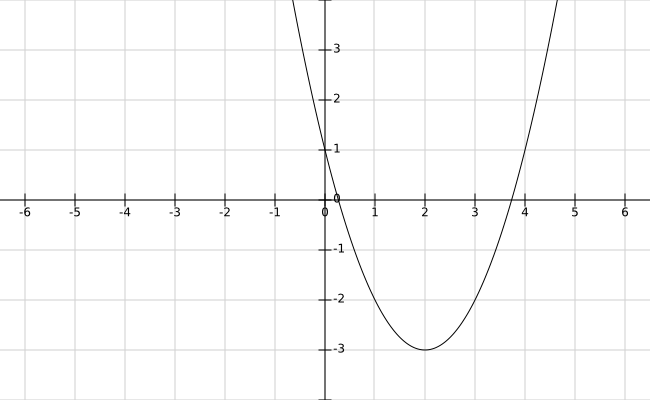## Section 2.6 Clicker Problems

1. The graph of $y=x^3$ is shifted 3 units down and 7 units left.
Which of these is an equation for the new graph?

A. $y=(x-3)^3-7$
B. $y=(x-7)^3-3$
C. $y=(x+7)^3+3$
D. $y=(x+7)^3-3$

2. A translation of the graph of $y=x^2$ is shown below. Which of these equations corresponds to the graph?

A. $y=(x+2)^2-3$
B. $y=(x-2)^2-3$
C. $y=(x-3)^2-2$
D. $y=(x+3)^2-2$3. Which of these describes the graph of $y=3+\sqrt{x-4}$ ?

A. The graph of $y=\sqrt{x}$ shifted down 4 and right 3
B. The graph of $y=\sqrt{x}$ shifted up 3 and right 4
C. The graph of $y=\sqrt{x}$ shifted up 3 and left 4
D. None of the above

4. The range of $f(x)=x^2$ is $[0,\infty)$. So, what is the range of $g(x)=x^2-5$?

A. $[5,\infty)$
B. $[-5,\infty)$
C. $[0,\infty)$
D. None of the above